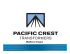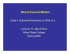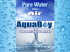# Optimal and economic selection of turn ratio for unit transformer

## Transcription

Optimal and economic selection of turn ratio for unit transformer
```Leonardo Electronic Journal of Practices and Technologies
Issue 26, January-June 2015
ISSN 1583-1078
p. 17-30
Optimal and economic selection of turn ratio for unit transformer using
generator capability curves of synchronous generators
Seyed M.H. NABAVI *, Somayeh HAJFOROOSH2, Sara SETAYESH3
1
Department of Electrical Engineering, Tabriz Branch, Islamic Azad University, Tabriz, Iran
2
Department of Electrical Engineering, Curtin University, Perth, WA, Australia
3
SAKOO Consulting Engineers, Tehran, Iran
*
*
E-mails: [email protected]
Corresponding author, phone: +61426446100
Abstract
One of the most important subjects in power systems is an optimal and
economic design of power transformers. In this paper, determination of turn
ratios for power transformers that are directly connected to generators is
evaluated considering reactive power generation and its impact on the optimal
turn ratio selection. A generator capability curve has been used to explain all
details. Three different methods have been examined and the simulation
results have been compared in a real test system, on Rudbar-Lorestan,
Karkheh, Karoon 3, Masjed Solayman, and Gotvand hydro power plants. The
obtained results illustrate that without considering limits of reactive power the
achieved turn ratios can be much higher than the optimal values.
Keywords
Turn ratio; Generator capability curves; Optimal and economic designing
Introduction
Recently, all industrial countries prefer to have an uninterrupted operation and
generation, and continuous transferring and distribution of power systems and increase the
17
Optimal and economic selection of turn ratio for unit transformer using generator capability curves …
Seyed M.H. NABAVI, Somayeh HAJFOROOSH, Sara SETAYESH
social welfare [1-6] and have reliable power . Transformers are the most important devices
to transfer and distribute in power systems. On the other hand, transformers are very
expensive and their halt may stop all other related equipment, which incurs plentiful damages.
Therefore, several optimization techniques have been studied in the literature.
Determination of optimal turn ratios of transformers that are directly connected to
generators is one of the important issues to design a power transformer . The study of
designing power transformers using different methods was established on [9-12]. In 2006, S.
Padma, R. Bhuvaneswari and S. Subramanian present a power transformer design
methodology using Simulated Annealing Technique . In 2012, A. Khatri, H. Malik and
O.P. Rahi, used genetic algorithm to find the optimal design of power transformer . Then,
a novel method of ampere-turn distribution is presented in  to optimize the distributions of
ampere-turns.
As mentioned in the above references the authors did not consider the impact of the
reactive power on the determination of the optimal turn ratios selection in transformers that
are directly connected to generators. This paper presents a precise and optimal calculation
strategy to calculate turn ratios for power transformers based on IEEE Standards. The results
of the presented algorithm are compared with the actual results that already achieved in Iran
for different hydro power plants. Furthermore, in order to compare precision of these results,
different calculation methods have been proposed [12-13]. These calculations are based on the
information from performance capability curve of Rudbar-Lorestan Hydro Power Station
.
In this paper, first the limits of reactive power generation in synchronous machines
have been presented. Next, the studies of different methods to calculate power transformers
turn ratios are discussed. Then, the results of the presented algorithm have been studied.
Material and method
Limits of reactive power generation in synchronous machines
In the study of voltage stability and long-term stability, limits of reactive power
generation by synchronous machines are important . Generator capability curves that
usually submitted by manufacturers indicate the active and reactive powers of a generator
18
Leonardo Electronic Journal of Practices and Technologies
Issue 26, January-June 2015
ISSN 1583-1078
p. 17-30
towards a system. When a generator is in over exciting condition, it generates reactive power;
and when it is in under exciting condition, it absorbs reactive power. This section studies
generator capability curves of synchronous machine to calculate the minimum and maximum
reactive power generation limits, which are the important parameters to select the optimal turn
ratio for a power transformer.
A.
Reactive power generation curves
Generally, nominal capacity of a synchronous machine can be indicated by MVA in a
specific voltage and power factor (usually 85-90% of lead) in which the synchronous machine
is able to work continuously without abnormal temperature increment. In addition, real output
power of the synchronous machine depends on turbine ability and nominal MVA machine
limits. Therefore, limitations of a generator should be known for the optimal utilization. Then,
the continuous generation of the reactive power will be limited by three criteria including
armature current limit, excitation current limit, and temperature limit at the end of the
armature. In the following sections, performance limitations of synchronous generators will
be presented .
B.
Armature current limit
Armature current limit incurs RI 2 losses and the related energy must be dissipated to
preserve conductors and environment temperature in limits (Figure 1- Curve #2).
In the selected generator, rated output power is 255000 kVA, rated voltage is 15750
V. In this figure, curves 1-6 are Maximum Field Current, Armature Current, Practical
Stability, Theoretical Stability, Minimum Field Current and Reluctance Power, respectively.
For a synchronous machine, the power is:
S = P + jQ = 3|Et||It|(cos(φ) + jsin(φ))
(1)
where, φ is power factor angle, Therefore in P-Q space the current limit of this armature will
be as a circle with MVA radius and origin of coordinates. In details, one of the generator
nominal capacity limitations is the maximum current that an armature can withstand without
exiting from its temperature limits. This limitation is shown in (Figure 1- Curve #2).
19
Optimal and economic selection of turn ratio for unit transformer using generator capability curves …
Seyed M.H. NABAVI, Somayeh HAJFOROOSH, Sara SETAYESH
Figure 1. Armature temperature limits
C.
Excitation current limit
The second limitation in operation of the synchronous machine can be due to the
temperature losses of current (RfdIfd2). The geometrical place of fixed exciting current is
obtained by using the steady state equivalent circuit in Figure 2. In addition, the equivalent
circuit of Figure 2 expresses relation between IA, EA and Vt if Xd = Xq= Xs, where X is the
leakage reactance; Xa is the armature reaction reactance, Xs = Xa + X is the synchronous
reactance; Ra = armature resistance; Vt is the terminal voltages; IA = armature Current, and EA
is the magnetizing voltage.
Xa
IA
Ra
+
EA
Vt
−
Figure 2. The equivalent circuit of steady state synchronous machine
According to equation (1), it can be seen that, for a given exciting current, relation
between real and reactive powers is a circle with Q = -3Et/XS and P=0 as a centre and -3EtIf/XS
as a radius. Therefore, the effect of maximum nominal current on power generation capability
20
Leonardo Electronic Journal of Practices and Technologies
Issue 26, January-June 2015
ISSN 1583-1078
p. 17-30
of this machine in P-Q space can be shown according to Figure 3. Regularly, the intersection
of temperature limits curve of armature and excitation indicates MVA capacity and nominal
power factor for generator. This point is indicated with “A” in Figure 3.
Field Current Heating Limit
X ad Et i fd
A (Rated MVA,p.f .)
XS
Et2
XS
Armature Current Heating Limit
Undervoltage 0.95 pu
Rated voltage 1.00 pu
Overvoltage
1.05 pu
Figure 3. Excitation limits of T synchronous generator
D.
Temperature limit at the end of armature
The third limitation of operation is the generated temperature at the end of the
machine armature. This limitation usually affects power generation curve of the synchronous
machine in under exciting condition of generator. More details about intersections of end area
of a synchronous generator are presented in . The flux of this area enters and exits
perpendicular to stator sheets. This causes to generate eddy currents, which produces heat at
the end of machine. In addition, strong exciting currents corresponding to over exciting
condition keep the spacer ring in saturation state to limit the end leak flux in small amount.
However, in an under-excitation state, field current is low and the spacer ring will not
be saturated. This increases leak flux of end area in armature. Also, in the under exciting
condition state, the flux of armature currents is aggregated with flux of exciting currents .
Therefore, the end flux is bounded, which applies limitation on generation capability of
synchronous machines with cylindrical rotors. This is shown in Figure 4, which includes the
21
Optimal and economic selection of turn ratio for unit transformer using generator capability curves …
Seyed M.H. NABAVI, Somayeh HAJFOROOSH, Sara SETAYESH
limit by temperature of armature current.
It should be mentioned that the temperature limits of exciting current and armature
current in P-Q space are depended on the armature voltage. Moreover, in terms of design
point of view, the mentioned limitations are applied based on the machine operation
capabilities. Thus, extra limitations may be applicable on the machine operation because of
the power system stability .
Q(p.u)
P(p.u)
Armature Current Heating Limit
End Regin Heating Limit
Figure 4. Temperature limits at the end of armature in a synchronous generator
E.
Synchronous generator capability curves drawing steps:
1. Select an origin on a paper and determine P and Q scales on it.
2. Draw a circle around the origin with radiuses |S| = 3|Et||It| equal to nominal
power of machine (KVA). This curve indicates temperature limit of the stator.
3. Draw a circle with a center of Q = -3Et/XS on Q axis and with radius of 3EtIf/XS. The curve indicates temperature limit of exciting current and
intersection of this curve with temperature curve of armature shows MVA
capacity and the nominal power of generator.
4. Draw a line from 60% point of maximum absorbable reactive power by the
generator to 120% point of the maximum generation reactive power that
22
Leonardo Electronic Journal of Practices and Technologies
Issue 26, January-June 2015
ISSN 1583-1078
p. 17-30
multiply to 1 as a power factor value.
5. Draw a parallel line to Q from P = 3|Et||It|cos(φ). This line indicates the
maximum generation active power of generator in overexciting condition .
Moreover, Figure 5 shows a typical capability curve for a synchronous generator in S
space, in which P is the horizontal axis and Q is the vertical axis. If S is inside the curve, the
machine neither is hot nor is out of its synchronous state.
Q
P = 3 Et I t Cosϕ
QMAX
3 Et I t
−3 Et I fd
1.2 × S
XS
0
0.2
0.4
0.6
0.8
1.0 1.2
P
QMin = −−%60
%60QQMAX
M ax
Q=
− 3 Et
XS
Figure 5. The performance capability curve for a synchronous generator in S plain
Study of different methods to calculate power transformers turn ratios
This section analyses and investigates different methods to calculate the power
transformer turns ratios.
A.
Method 1
In this method, turn ratios and the secondary voltage are obtained by using equation
(2) as follows :
Ua = Ur(1+Uφ/100)
(2)
Uφ = Uφ' + 1.2(Uφ2/100)
(3)
Uφ' = URrcos(φ) + UXrsin(φ)
(4)
Uφ'' = URrsin(φ) - UXrcos(φ)
(5)
where,
where, cos(φ) is Nominal power factor; Pkr is Total losses; Sr is Nominal power; Ukr is Short-
23
Optimal and economic selection of turn ratio for unit transformer using generator capability curves …
Seyed M.H. NABAVI, Somayeh HAJFOROOSH, Sara SETAYESH
circuit voltage (%), and Ur is Nominal voltage.
It should be noted that, in this method the limits to generate reactive power for the
generator connected directly to the transformer are not considered which cause inaccurate
results.
B.
Method 2
Figure 6 shows different parameters, which are used, in input information. In this
method, the turn ratio and the secondary voltage can be obtained by solving equations (6) and
(7) simultaneously, as follow :
Figure 6: Parameters for input information
V2cos(δ) = 1 + RPU[cos(δ - φ)] + X[cos(δ + 90 - φ)]
(6)
V2sin(δ) = RPU[sin(δ - φ)]+X[sin(δ + 90 - φ)]
(7)
where,
SB: Nominal power (MVA)
VB: Per Unit voltage (kV)
IB: Per Unit nominal current (A)
ZB: Per Unit impedance, this is obtained from ZB=VB2/SB
PSCL: Short-circuit loss in full load (kW)
RP.U: Resistance per unit, which is obtained by RP.U=PSCL2/3IB2
Uk = X%: Short-circuit voltage (%)
cos(φ): Nominal power factor
It should be noted that this method is not also precise due to not-considering
limitations of reactive power generation to calculate the turn ratio.
C.
Method 3
To calculate an accurate and practical turn ratio, considering reactive power
generation/absorbing limits by the system generators are important. Different steps to
calculate an optimal turn ratio for a power transformer using presented algorithm are:
24
Leonardo Electronic Journal of Practices and Technologies
Issue 26, January-June 2015
ISSN 1583-1078
p. 17-30
Step 1: Drawing VS - Q curve based on using equation (8) :
Vs
⎡
⎤
⎢ MW ± jMVAR ⎥
THV ⎤ ⎢
⎥
MVAT
= Vg VgBase ⎡⎢ V
−
⎥
⎢
⎥ (RT +
TLV
V
V
⎛
⎞
⎦
⎣
gBase
⎢ V ⎜
⎟ ⎥
g ⎜ VTLV ⎟
⎢⎣
⎝
⎠ ⎥⎦
(8)
jXT )VTH
where VS is the system voltage (kV); Vg is Generator voltage (per unit); VgBase is Nominal
voltage (kV); VTHV is Maximum acceptable voltage of system; VTLV is Minimum acceptable
voltage of system; Rr is Transformer resistance in nominal voltage and power; Xr is
Transformer reactance in nominal voltage and power; cos(φ) is Generator’s power factor;
QMIN is Maximum reactive power absorbed by generator; QMAX is Maximum reactive power
produced by generator, and MVAT is Nominal output power of generator.
Step 2: Drawing the capability curve.
Step 3: Calculation of QMIN and QMAX by using the performance capability curve of
generator Figure 6.
Step 4: Draw two parallel vertical lines starting from QMIN and QMAX.
Step 5: Find the intersections of the vertical lines (obtained from Step 4) with the VS Q curve.
It should be noted that the obtained points from the intersection of parallel lines and VS
- Q curve, represents the minimum and maximum system voltage when the reactive power is
absorbed or generated. These points are shown in Figure 7.
Results
Analyzing the curves for the Rudbar-Lorestan hydro power station
The input parameters for Rudbar-Lorestan power station plant are shown in Table 1.
VS
400kV
Vg
1 pu
Table 1. Parameters for Rudbar-Lorestan power station plant
Xr %
VgBase
VTHV
VTLV
Rr
cos(φ)
0
14
0.9
15.75kV 420kV 380kV
MVAT
255
25
Optimal and economic selection of turn ratio for unit transformer using generator capability curves …
Seyed M.H. NABAVI, Somayeh HAJFOROOSH, Sara SETAYESH
QMIN
QMAX
Figure 7. QMIN and QMAX for the Rudbar-Lorestan generator
Regarding to the input values and performance capability curve, QMIN and QMAX are
equal to -122.4 and +121.1, respectively. These points are shown in Figure 8.
In following, equation 8 based on step 1 and in order to calculate the Rudbar-Lorestan
Power Station plant is simulated by MATLAB software. The simulation results are shown in
Figure 8.
Study of different assumable states for turn ratios to calculate the optimal value
With considering the obtained values of QMIN and QMAX, the VS - Q diagram will be as
Figure 8. In addition, four steps for turn ratios of power transformers are considered in this
diagram. In this Figure, lines A-E, B-F, C-G, D-H show the turn ratios of 410/15.75,
400/15.75, 390/15.75, and 380/15.75 respectively.
According to these lines, it seems that in turn ratios of 420/15.75, 380/15.75 the
minimum voltage level which is happened in reactive power absorption state is lower than the
standard level (380kV).
Also, in the turn ratios of 420/15.75 the minimum and maximum value of voltage
levels have a large difference from standard values (380kV to 420kV) and the selection of this
turn ratios causes increase in protective equipment expenses. Therefore, this ratio is not
optimal economically. However, there will not be the economic and technical limitations by
selecting 410/15.75 as a turn ratio.
26
Leonardo Electronic Journal of Practices and Technologies
Issue 26, January-June 2015
ISSN 1583-1078
p. 17-30
480
470
460
420
15.75
450
440
H
410
15.75
G
F
Voltage Level (kV)
430
420
410
E
400
390
380
370
D
C
B
360
350
380
15.75
400
15.75
A
340
330
- 220- 200- 180- 160- 140- 120- 100 - 80 - 60 - 40 - 20
0
20
40
60
80 100 120 140 160 180 200 220
Reactive Power (Mvar)
Figure 8. VS - Q curve for Rudbar-Lorestan power station plant
The reason is that voltage level in point C is higher than minimum voltage level
(380kV), and it is higher than maximum voltage level in point G.
It should be noticed that in point C, the minimum voltage level is chosen more than
380kV; the reason is considering 10% error for the transformer impedance, which this error
level is chosen based on IEC60076 standard.
IN addition, in order to show the correctness and accuracy of the proposed algorithm,
simulations have been done to calculate the turn ratios of Karkheh , Karoon 3, Masjed
Soleyman , and Gotvand  projects. The results are shown in Table 2.
Table 2. Results of first and second method and their comparison with the results of the
proposed algorithm
Plants name
Karkheh
Karoon 3
Masjed Soleyman
Gotvand
Rudbar-Lorestan
Method 1
423
427
427
422
427
Method 2
421
425
423
419
417
Proposed algorithm
411
409
409
410
410
Implemented values
410
410
410
410
410
Moreover, the results of the first and the second methods are presented to compare
with. Studying of these three methods results show that not considering reactive power
27
Optimal and economic selection of turn ratio for unit transformer using generator capability curves …
Seyed M.H. NABAVI, Somayeh HAJFOROOSH, Sara SETAYESH
generation limitations for a generator connected to a transformer causes error in calculation of
turn ratios.
Conclusions
Regarding to the importance of transformers in transmission systems, designing and
selection of transformers’ parameters play an important role for optimal power transferring.
Therefore, the optimal transformer turn ratios selection is essential and necessary. In this
paper, different methods for optimal and economic designing of power transformers turn
ratios are studied and the results are compared.
The investigation shows that not considering the reactive power generation limits in
generator connected to the transformer cause arousing error in turn ratios calculation. In this
paper, the impact of considering reactive power limits has been studied and tested for
different real hydro power plants. Comparison between the obtained results based on the
suggested algorithm and the real values shows the accuracy and precision of this method.
References
1.
Nabavi S. M. H., Hajforoosh S., Hajforosh S., Karimi A., Using genetic algorithm for
social welfare maximization in deregulated power markets by optimal locating and
sizing of TCSC, Int Rev Model Simul, p.1447–53, 2010.
2.
Masoum M. A. S., Nabavi S. M. H., Kazemi A., Social welfare maximization in doublesided auction market by placement and sizing of TCSC using fuzzy-based genetic
algorithm, International Review of Electrical Engineering, 5(5), p. 2392- 2404, 2010.
3.
Nabavi S. M. H., Kazemi A., Masoum M. A. S., Social Welfare Improvement by TCSC
using Real Code Based Genetic Algorithm in Double-Sided Auction Market, Advances
in Electrical and Computer Engineering, 2011, 11, no. 2, p. 99-106,.
4.
Nabavi S. M. H., Hajforoosh S., Masoum M. A. S., Placement and sizing of distributed
generation units for congestion management and improvement of voltage profile using
particle swarm optimization, Innovative Smart Grid Technologies Asia (ISGT), p.1-6,
28
Leonardo Electronic Journal of Practices and Technologies
Issue 26, January-June 2015
ISSN 1583-1078
p. 17-30
2011.
5.
Hajforoosh S., Nabavi S. M. H., Masoum M.A.S., Coordinated aggregated-based
particle swarm optimisation algorithm for congestion management in restructured power
market by placement and sizing of unified power flow controller, Science, Measurement
& Technology, IET, p.267-278, 2012.
6.
Hajforoosh S., Nabavi S. M. H., Masoum M. A. S., Optimal Particle Swarm Based
Placement and Sizing of Static Synchronous Series Compensator to Maximize Social
Welfare, Journal of Electrical Engineering & Technology, p. 501-512, 2012.
7.
Nabavi S. M. H., Gholami A., Kazemi A., Masoum M. A. S., Evaluation of leakage
current measurement for site pollution severity assessment, Leonardo Electron. J. Pract.
Technol, p. 39–54, 2007.
8.
IEEE Guide for Transformers Directly Connected to Generators, Revision of IEEE Std
C57.116-1989, p.1-48, 2014.
9.
Padma S., Bhuvaneswari R., Subramanian S., Optimal Design of Power Transformer
Using Simulated Annealing Technique, IEEE International Conference on Industrial
Technology, p.1015-1019, 2006.
10. Khatri A., Malik H., Rahi O. P., Optimal Design of Power Transformer Using Genetic
Algorithm, International Conference on Communication Systems and Network
Technologies, 2012, p.830-833.
11. Feng B., Wang G.L., Liu H. D., Zhang H. J., Optimum Design of Ampere-turn Balance
for Power Transformer, IEEE International Conference on Applied Superconductivity
and Electromagnetic Devices (ASEMD), p.387-390, 2013.
12. Chapman, S., Electric machinery fundamentals. Tata McGraw-Hill Education, 2005.
13. Hawary M. E. El., Principles of electric machines with power electronic applications,
IEEE Press, 2002.
14. Iran Water and Power Resources, Development Company, Technical specification for
Rudbar-e-Lorestan Dam and Power Plant, 2011, URL: http://en.iwpco.ir/Rudbar/default.aspx.
15. Sauer P.W., “Power System Dynamics and Stability”, Handbook of Electrical Power
System Dynamics: Modeling, Stability, and Control, 2013.
16. Iran Water and Power Resources, Development Company, Technical specification for
Karoon 3 Dam and Power Plant, 2011, URL: http://en.iwpco.ir/IWPCO.aspx.
17. Iran Water and Power Resources, Development Company, Technical specification for
29
Optimal and economic selection of turn ratio for unit transformer using generator capability curves …
Seyed M.H. NABAVI, Somayeh HAJFOROOSH, Sara SETAYESH
Karkheh Dam and Power Plant, 2004, URL: http://jahadtossee.ir/company-manabeab-summarycompleted-en.html.
18. Iran Water and Power Resources, Development Company, Technical specification for
Gotvand Dam and Power Plant, 2006, URL: http://en.iwpco.ir/Gotvand/default.aspx.
19. Iran Water and Power Resources, Development Company, Technical specification for
Masjed
Soleyman
Dam
and
http://www.moshanir.co.ir/en/masjed.aspx.
30
Power
Plant,
2001,
URL:
```

### Type VOZ-20 Voltage Transformer (English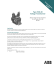### EE 328 _lect_03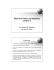### Sprint Enclosure Manual - Inverter Drive Supermarket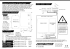### HYDRO POWER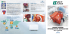### 2015 SECME Olympiad Generator Section Corrections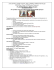### EEE307 Electromechanical Energy Conversion Homework I (Due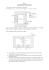### - Netico GmbH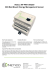### Medford, Oregon